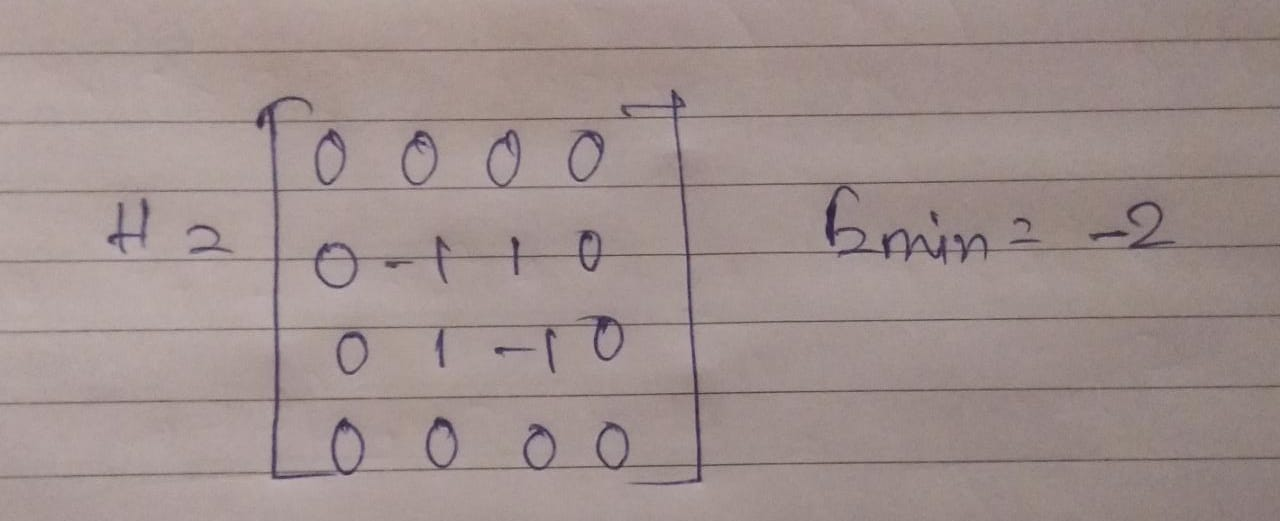# Hamiltonian to QUBO conversion and vice versa

I have a Hamiltonian. How can I transform the Hamiltonian to QUBO? Is there any other way of doing the reverse(QUBO to hamiltonian)?

• Is your Hamiltonian in Ising form? If so, you can submit it directly to the QPU with the sample_ising() function in Ocean.

Thanks!

•this is my hamiltonian. Solving with this with Numpy or analytically gives the minimum eigen value of -2, which is correct. Solving this with a gate model VQE algorithm also gives -2. how can i solve(find the minimum energy) this in DWAVE machine?

* This hamiltonian is a 2 qubit hamiltonian (4x4 matrix). What will the the dimension of QUBO matrix of this hamiltonian?

* Is it possible to transform every hamitonian to QUBO and vice versa? If possible please elaborate the mathematical steps.

• I have had good luck with converting Hamiltonians to Ising and QUBO forms using PyQUBO., where the Hamiltonian is in the form of a mathematical expression. The open source project is on Github at: https://github.com/recruit-communications/pyqubo .

• As per my knowledge if a hamiltonian is in Ising format it can be transformed in QUBO and vice versa using this PyQUBO. How can i be sure that the hamiltonian i mentioned above is an "Ising hamiltonian"?

Operator decomposition of the above hamiltonian follows

`H = 0.5XX + 0.5YY + 0.5ZZ - 0.5IIWhere X,Y,Z are pauli matrices and I is the Identity matrix`
• If it is not an Ising hamiltonian then is it possible to transform the hamiltonian into an Ising hamiltonian?
• How to transform a hamiltonian to Ising hamiltonian?
• Hello,

I have discussed this with my colleagues and have collected some information for you.

When people talk about converting between Ising and QUBO they are talking about a classical Ising Hamiltonian.

This transformation is by a simple change of variables.

The quantum Hamiltonian we work with is a transverse field Ising model with 1- and 2-body Z terms and 1-body X terms.

At the end of the anneal (s=1) the transverse field (and therefore the X-basis terms) goes to zero, and we have a classical Ising Hamiltonian that can be translated into QUBO.

In light of this, we can see that the idea of translating to QUBO in the middle of the anneal doesn't make sense.

The 2-qubit Hamiltonian you show is a quantum Hamiltonian acting on a 2-qubit Hilbert space.

A 2-qubit classical Ising Hamiltonian is in 2 dimensions rather than 4, and has the form H(x) = x' J x + h' x.

This can be translated into QUBO form with the affine transformation y = (x+1)/2, with a cost function C(y) = y' Q y.

For quantum Hamiltonians, some but not all can be expressed as a transverse field Ising model.

For example, the 4x4 matrix you shared cannot be expressed in the given basis because the off-diagonal entries do not correspond to a uniform transverse field.

I hope this proves to be helpful!

• Thank you David J for your attention. This is the answer i was looking for.

• Great! Glad we could be of assistance!
Feel free to reach out with any other questions you might have.